# 突破正则匹配：探寻SQL注入绕过WAF的本源之道

0X01 WAF介绍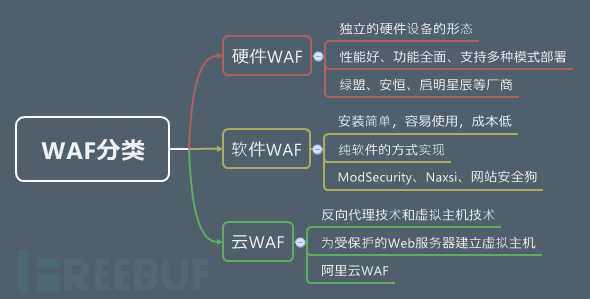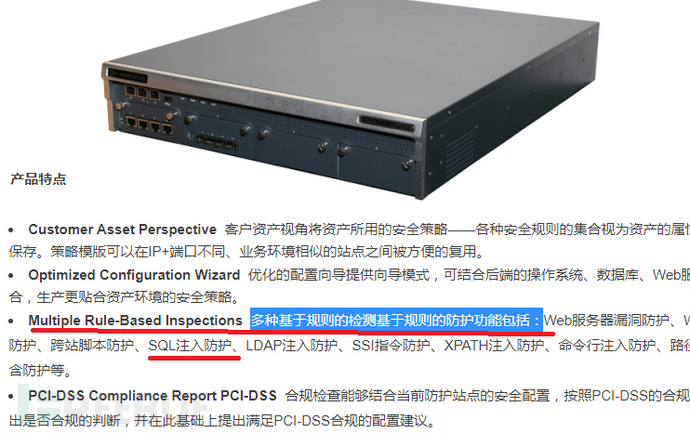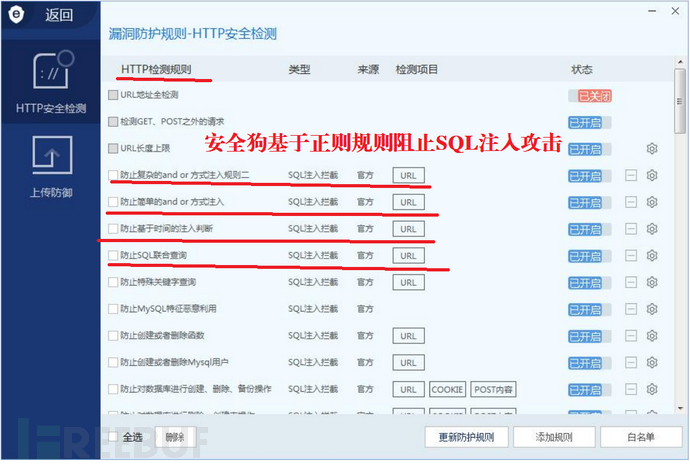WAF：腾讯云Web防火墙中对于防止SQL注入的描述是基于AI+规则，说明云WAF在防护SQL注入时依然离不开基于规则的正则匹配。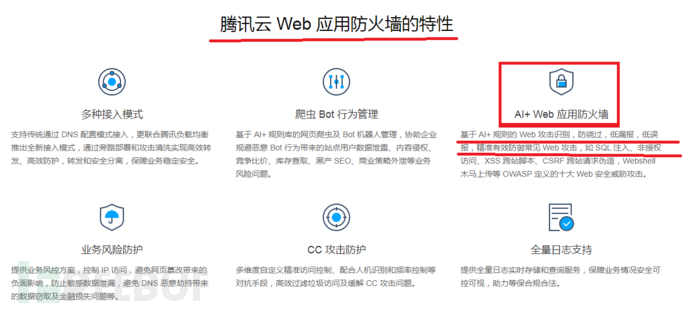0X02 WAF正则匹配原理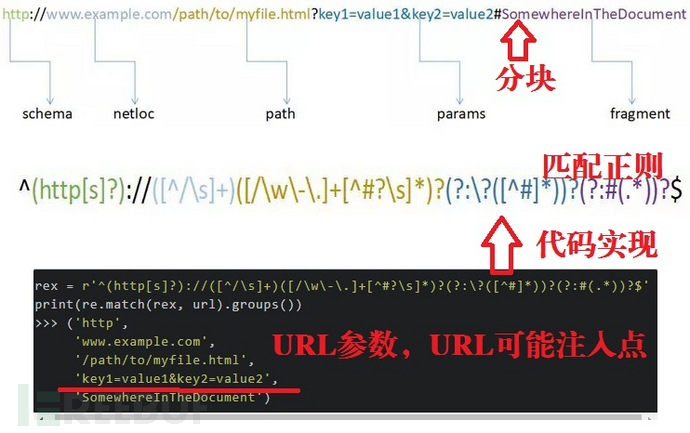"[\x22\x27]\s*OR|AND\s*\d+\x3d\d+"

"(OR|AND)(\s+?).+?=[\x22\x27](\s+?)[\x22\x27]\$"

"(OR|AND)(\s+?).+?=(\s+?)[\x22\x27]\$", "([\x22\x27])?(\s+?)--(\s+?)([\x22\x27])?"

".+?=[\x22\x27]\*[\x22\x27]\s(AND|OR)\s.+?=[\x22\x27][\x22\x27]", "\x3bDROP"

"((\x27)|(\x22))\*((\x27)|(\x22))"

"#.+?WHERE.+?SELECT", "--.+?[\x22\x27]","%27%20","\/\*|\*\/",";.+?\$"

"\w*((\%27)|(\'))(\s?)((\%6F)|(\%4F))((\%72)|(\%52))"

"\w*((\%27)|(\'))(\s?)((\%6F)|o|(\%4F))((\%72)|r|(\%52))"

"\w*((\%6F)|(\%4F))((\%72)|(\%52))(\s?)((\%27)|(\'))",".+?(\%2A).+?\$"

".+?(0x3(a|A)).+?\$", ".+?information_schema.+?\$"

0X03 从正则匹配角度反观WAF绕过手法